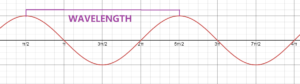# Optical wavelength to frequency converter

While in optics wavelength are mostly used to define the light signal, usage of frequency of a laser for example is not uncommon. Both properties are related, in order to jungle more easily between them you can use below calculator.

## What is the relation between frequency and wavelength ?

Light is a electromagnetic radiation that behave like a wave. One important characteristic of  waves is their frequency.

Frequency is defined as the number of times a crest of the signal occurs in a given time.

Wavelength refers to the distance between two crests of the signal.

Both are related to each other following this equation : Frequency = Speed of the signal / Wavelength

In optics, the signal is by definition light, as the speed of light is known ( c = 299,792,458 m/s), the equation can be simplified in :

F=c/λ

F : Frequency (Hz)     c : speed of light in vacuum  (m/s)  λ (Lambda) : wavelength (m)## When using frequency or wavelength ?

As explained above both values are related, so they can both be used for defining an optical signal as they convey the same information.

Usage of one or an other often depends on the context and application. When comparing light to other signals (for communication purposes for example) frequency will be preferred as it is proportional to the amount of information that can be transferred. Whereas, for optical application in UV, Visible or IR wavelength will be preferred as it is directly related to the human perception of the signal.